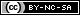# MathsFaculty

A place for maths teachers, just like you, to share original resources.

## Multiplying and Dividing Decimals#### Resource Type: Worksheet - Print

A basic drill sheet for multiply and dividing decimals includes:

• multiplying decimals by 10, 100, 1000
• dividing decimals by 10, 100, 1000
• multiplying decimals by any numbers
• dividing decimals by a whole number
• dividing decimals by a decimal

Attribution-Noncommercial-Share Alike